Test: Periodic Properties- 2

# Test: Periodic Properties- 2

Test Description

## 30 Questions MCQ Test Inorganic Chemistry | Test: Periodic Properties- 2

Test: Periodic Properties- 2 for Chemistry 2022 is part of Inorganic Chemistry preparation. The Test: Periodic Properties- 2 questions and answers have been prepared according to the Chemistry exam syllabus.The Test: Periodic Properties- 2 MCQs are made for Chemistry 2022 Exam. Find important definitions, questions, notes, meanings, examples, exercises, MCQs and online tests for Test: Periodic Properties- 2 below.
Solutions of Test: Periodic Properties- 2 questions in English are available as part of our Inorganic Chemistry for Chemistry & Test: Periodic Properties- 2 solutions in Hindi for Inorganic Chemistry course. Download more important topics, notes, lectures and mock test series for Chemistry Exam by signing up for free. Attempt Test: Periodic Properties- 2 | 30 questions in 90 minutes | Mock test for Chemistry preparation | Free important questions MCQ to study Inorganic Chemistry for Chemistry Exam | Download free PDF with solutions
 1 Crore+ students have signed up on EduRev. Have you?
Test: Periodic Properties- 2 - Question 1

### Incorrect order of ionic size is:

Detailed Solution for Test: Periodic Properties- 2 - Question 1

In a given lanthanide series , ionic radii decreases due to lanthanoid contraction . So size of Eu3+ should be larger than Gadolinium ion Gd3+

Hence option A is the answer.

Test: Periodic Properties- 2 - Question 2

### Among NaF , NaCl , NaBr and Nal , the NaF has highest melting point because

Test: Periodic Properties- 2 - Question 3

### Consider the following changes: M (s) → M (g)     ........(1) M (s) → M2+ (g) + 2e-   .......(2) M (g) → M+ (g) + 2e-   .......(3) M+ (g) → M2+ (g) + e-  .......(4) M (g) → M2+ (g) + 2e- .......(5)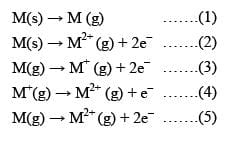The second ionization energy of M could be calculated from the energy values associated with:

Detailed Solution for Test: Periodic Properties- 2 - Question 3

M (s) → M (g)     ........(1)
M (s) → M2+ (g) + 2e-   .......(2)
M (g) → M+ (g) + 2e-   .......(3)
M+ (g) → M2+ (g) + e-  .......(4)
M (g) → M2+ (g) + 2e- .......(5)

As we are interested in 2nd I.E.
i.e. Energy required to form M2+ (g) from M+ (g)
∴ 4 is correct.
but this option doesn't exist.
⇒ 1 + 3 + 4 is also wrong
1 + 5 gives total energy for M(g) → M2+ (g)
∴ it is also wrong.
3rd reaction is wrong,
∴ 2 - 1 + 3 also dismised.
⇒ (d) is correct

Test: Periodic Properties- 2 - Question 4

The correct order of second I. E. of C, N, O and F are in the order:

Test: Periodic Properties- 2 - Question 5

Which is the correct order of ionization energies?

Test: Periodic Properties- 2 - Question 6

Which of the following statements is incorrect:

Test: Periodic Properties- 2 - Question 7

First ionization energy is the lowest with:

Test: Periodic Properties- 2 - Question 8

The incorrect statement among the following is:

Detailed Solution for Test: Periodic Properties- 2 - Question 8

I.E.2 of Na is higher than that of Mg because in case in Na, the second e has to be removed from the noble gas core while in case of magnesium removal of second e- electron gives a noble gas core. Mg has higher first ionization potential than Na because of its stable ns2 configuration.

Test: Periodic Properties- 2 - Question 9

The correct values of ionization enthalpies (in kJ mol–1) of Si, P, Cl and S respectively are:

Test: Periodic Properties- 2 - Question 10

The third ionization energy is maximum for:

Detailed Solution for Test: Periodic Properties- 2 - Question 10

Nitrogen has highest third ionization energy due to charge density.  As when the three electrons are remove from the gaseous state then boron have fully filled orbital that's why more energy is needed to remove the electron so more is ionisation energy.

Test: Periodic Properties- 2 - Question 11

Consider the following changes
A → A+ + e- : E1
and A+ → A2+ + e- ; E2
The energy required to pull out the two electrons are E1 and E2 respectively. The correct relationship between two energies would be...

Test: Periodic Properties- 2 - Question 12

The incorrect statement is:

Test: Periodic Properties- 2 - Question 13

First the ionization energies (in kJ/mol) of three representative elements are given below: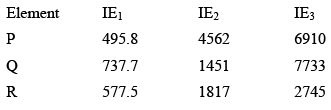Then incorrect option is:

Test: Periodic Properties- 2 - Question 14

Which of the following statement is correct regarding following process: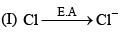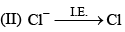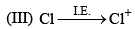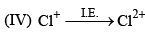Test: Periodic Properties- 2 - Question 15

The correct order of increasing electron affinity of the following elements is:

Detailed Solution for Test: Periodic Properties- 2 - Question 15

Electron affinity generally becomes more negative on moving from left to right along a period. It is because on moving across a period, the atomic size decreases.

As a result, the force of attraction exerted by the nucleus on the electron increases.

Consequently, the atom has a greater tendency to gain an electron. Hence, electron gain enthalpy becomes more negative.

Cl has more negative electron gain enthalpy than fluorine because in it the added electron goes to the smaller energy shell(n=2) and hence suffers significant repulsion from the electrons already present in this shell. And fluorine also possesses high charge density.

Test: Periodic Properties- 2 - Question 16

The second electron gain enthalpies (in kJ mol–1) of oxygen and sulphur respectively are:

Test: Periodic Properties- 2 - Question 17

Which of the following statements is correct:

Test: Periodic Properties- 2 - Question 18

Which one of the following statements is incorrect:

Detailed Solution for Test: Periodic Properties- 2 - Question 18

Electron gain enthalpy is the energy released when an electron is added to an isolated gaseous atom.

Its value is negative and the ease with which an atom accepts electrons its electron gain enthalpy becomes more negative that is, its value increases.

With an increase in nuclear charge, the electron gain enthalpy also increases.

Due to the extra stability of nitrogen (it has half-filled valence shell [He]1s22p3, which provides extra stability to it), it is very difficult to add an electron to it.

Therefore, it has almost zero electron gain enthalpy.

In general, on moving down the group, the electron gain enthalpy decreases due to an increase in the atomic size, but on moving from F to Cl the electron gain enthalpy increases.

Due to the very small size of F atom and absence of 3rd shell, the incoming electron experiences repulsion due to the valence electrons and its electron gain enthalpy is less than Cl. After Cl the electron gain enthalpy follows the general order and decreases on moving down to Br and I.

Test: Periodic Properties- 2 - Question 19

The formation of the oxide ion O2–(g) requires first an exothermic and then an endothermic step as shown below: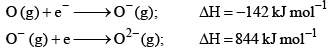This is because:

Test: Periodic Properties- 2 - Question 20

Identify the wrong sequence of the elements in a group

Test: Periodic Properties- 2 - Question 21

The electron affinity of the following elements can be arranged:

Detailed Solution for Test: Periodic Properties- 2 - Question 21

Electron affinity represents tendency of an atom to accept an electron. Electron affinity increases from left to right and decreases down the group. The images attached compares the electron affinity values of elements.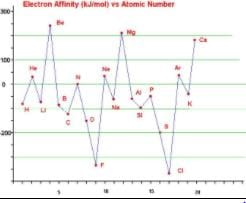Test: Periodic Properties- 2 - Question 22

In which of the following arrangements, the order is not correct according to the property indicated against it:

Test: Periodic Properties- 2 - Question 23

Which of the following statements is/are wrong:

Detailed Solution for Test: Periodic Properties- 2 - Question 23

All isoelectronic ions belong to the same period of the periodic table, is a wrong statement as F− and Na+ are isoelectronic but they belong to different periods.

Test: Periodic Properties- 2 - Question 24

Consider the following conversions: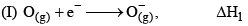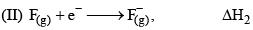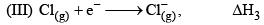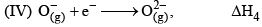That according to given informat ion the incorrect statement is:

Test: Periodic Properties- 2 - Question 25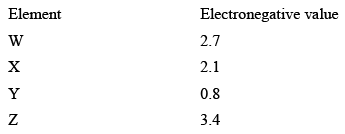The incorrect statement regarding given information is

Test: Periodic Properties- 2 - Question 26

In the compound M—O—H, the M—O bond will be broken if:

Test: Periodic Properties- 2 - Question 27

An element has an atomic number of 15 with which of the following elements will it show similar chemical properties.

Test: Periodic Properties- 2 - Question 28

If the ionization and electron gain enthalpy of an element are 275 and 86 Kcalmol−1 respectively, then the electronegativity of the element on the Mulliken scale is:

Detailed Solution for Test: Periodic Properties- 2 - Question 28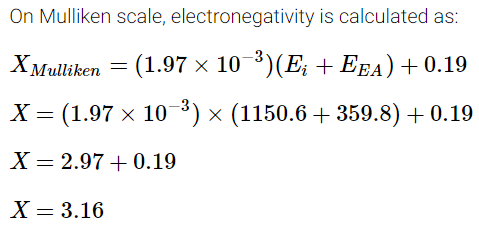Test: Periodic Properties- 2 - Question 29

Consider the following statements: (I) The radius of an anion is larger than that of the parent atom. (II) The ionization energy generally increases with increasing atomic number in a period (III) The electronegativity of an element is the tendency of an isolated atom to attract an electron.Which of the above statements is/are correct:

Test: Periodic Properties- 2 - Question 30

Which of the following order is correct for the property ment ioned in brackets:

## Inorganic Chemistry

36 videos|71 docs|16 tests
 Use Code STAYHOME200 and get INR 200 additional OFF Use Coupon Code
Information about Test: Periodic Properties- 2 Page
In this test you can find the Exam questions for Test: Periodic Properties- 2 solved & explained in the simplest way possible. Besides giving Questions and answers for Test: Periodic Properties- 2, EduRev gives you an ample number of Online tests for practice

## Inorganic Chemistry

36 videos|71 docs|16 tests